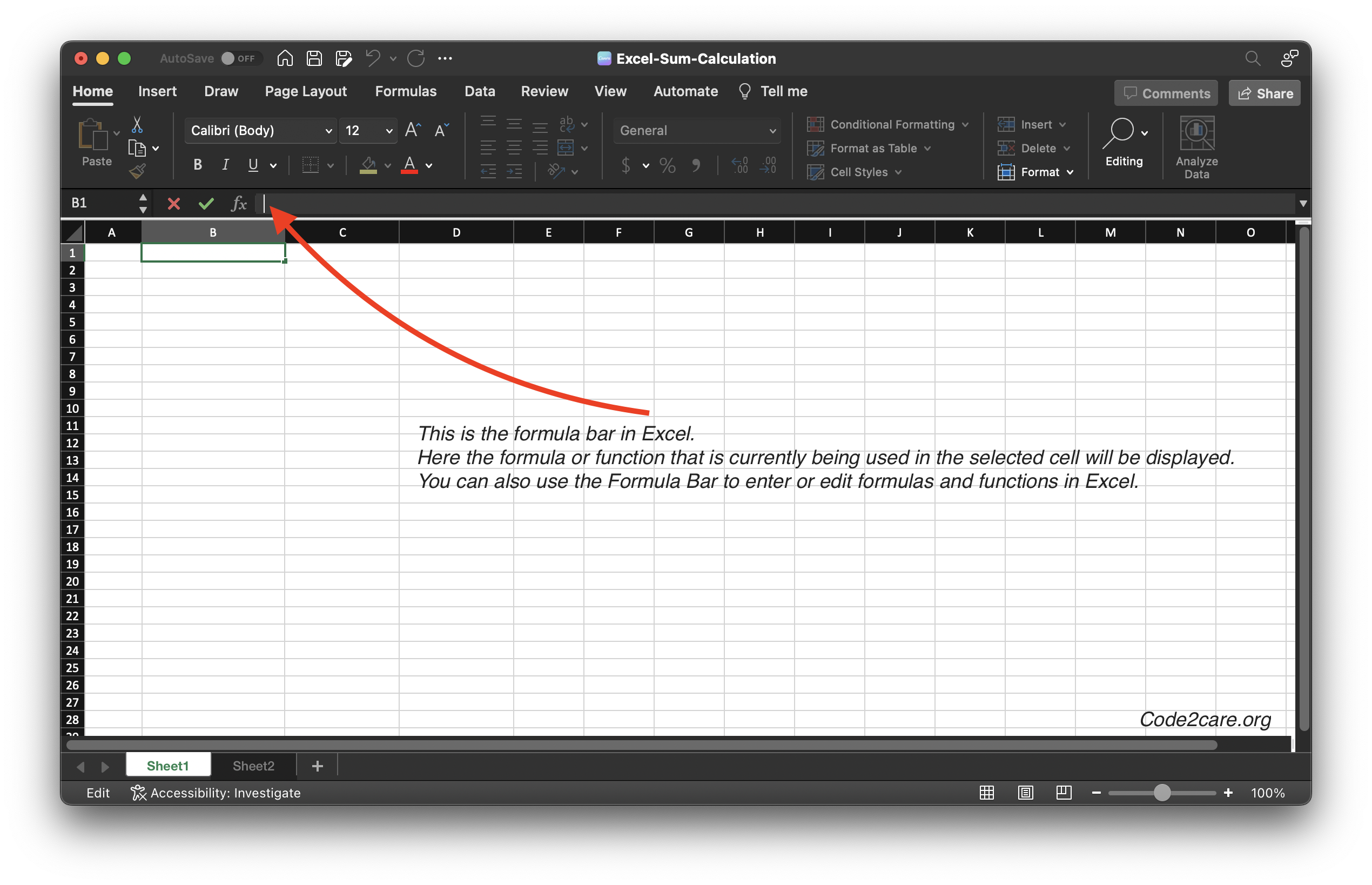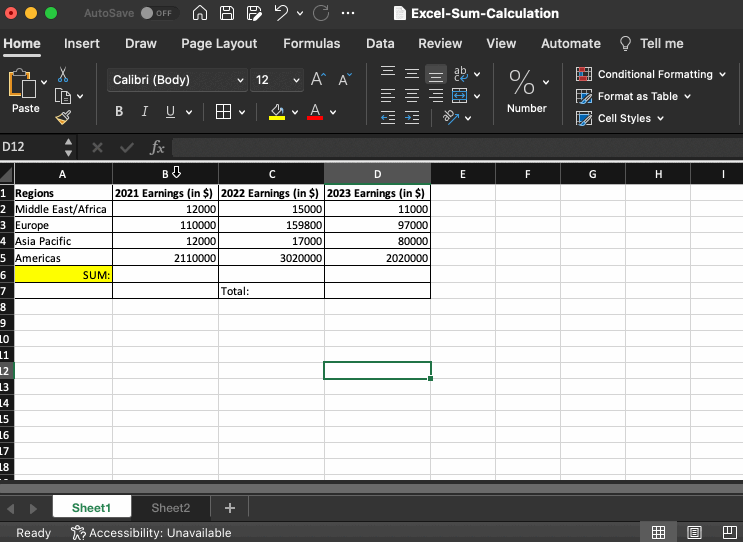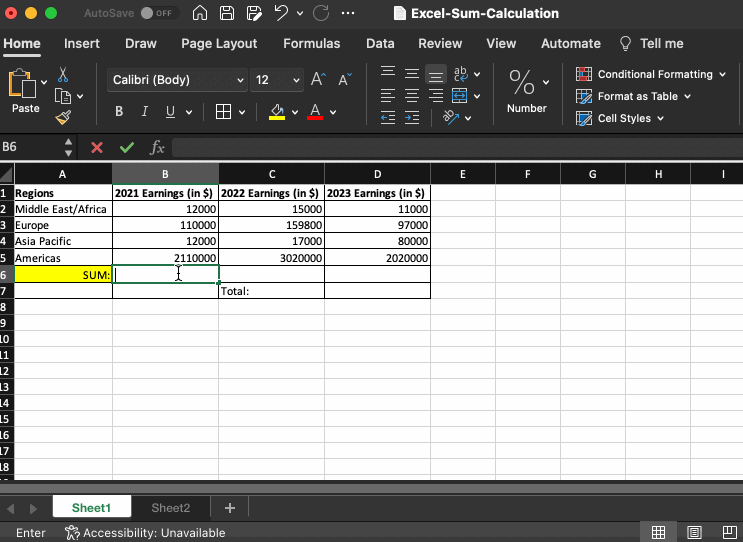# How to calculate SUM in Excel on Mac with Examples

Performing calculations, such as adding up a list of numbers, is one of the most common tasks in Microsoft Excel. Luckily, Excel provides many built-in functions to help you perform calculations quickly and easily. One of the most useful functions for adding up numbers is called SUM.

When you open an Excel sheet or create a new one on your Mac, you will notice a bar just below the ribbon labeled as fx this is called the "Formula Bar". This bar displays the formula or function that is currently being used in the selected cell. You can also use the Formula Bar to enter or edit formulas and functions in Excel.To learn how to calculate SUM, let us add some data to work with in Sheet1.

Example Data:
Region 2020 Earnings (in \$) 2021 Earnings (in \$) 2022 Earnings (in \$)
Region 12000 15000 11000
Region 110000 159800 97000
Region 12000 17000 80000
Region 2110000 3020000 2020000

## 1. Display SUM on the Status bar by selecting ColumnAs you can see in the above gif example, as you select Columns B, C, or D, the SUM is calculated and displayed on the bottom Status Bar in Excel. This is really useful when you just want to see how the numbers add up in different cells on the fly.

## Calculate SUM using SUM FormulaAs you would see in the above gif example, we have made use of the SUM formula in 3 ways.

### 1. By using the SUM function on the Cell

Move your pointer to the cell where you want to calculate the SUM, now type equal-to followed by SUM and open bracket, and select all the cells from column B to calculate.

``=SUM(B2:B5)``

### 2. By Click on the fx bar

When you click on fx you will see that a Formula Builder sidebar appears where you can click on SUM after making sure your position on the sheet is where you want to display the sum

``=SUM(C2:C5)``

### 3. Using the Formula Bar

Like example 1, we type the formula on the formula bar instead of the cell.

``=SUM(D2:D5)``

``````Note: All examples are based on Microsoft Excel for Mac running on macOS

Specifications:
Excel Version: Version 16.70 (23021201)
Operating System: macOS Ventura 13
Chip: Apple Silicon M2
Device: Macbook Pro``````
-

### Facing issues? Have Questions? Post them here! I am happy to answer!Author Info:

Rakesh is a seasoned developer with over 10 years of experience in web and app development, and a deep knowledge of operating systems. Author of insightful How-To articles for Code2care.